# How to derive custom SOA diagrams for power switches? – KBA234645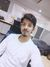Community ManagerCommunity Manager# How to derive custom SOA diagrams for power switches? – KBA234645

Community Translation: 電源スイッチのカスタムSOAダイアグラムはどのように導き出しますか？ - KBA234645

Version: **

The safe operating area (SOA) of power devices describes the operation conditions in which the safe operation of the device is guaranteed. This means that exceeding the operation conditions marked by the diagram lines can result in the breakdown of the device.

For basic and advanced understanding of the SOA diagram, see the Linear Mode Operation and Safe Operating Diagram of Power-MOSFETs app note.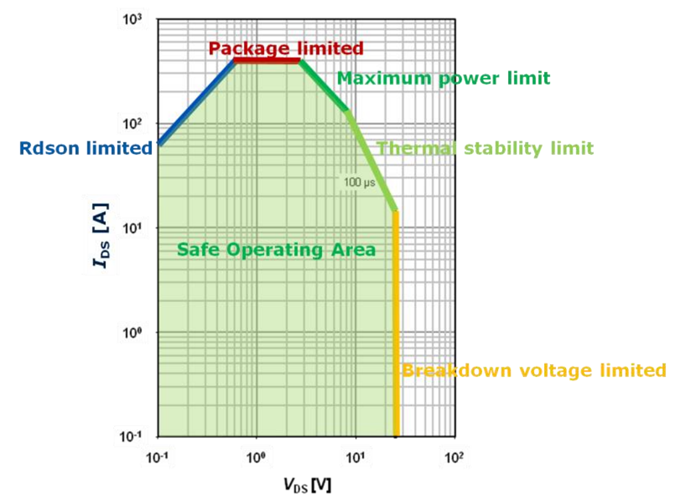This KBA is intended for those who need a SOA diagram in different conditions than that of the ones given in the datasheet. This article references the five different limitations (marked in colors). Therefore, an understanding of these is required for the understanding of the contents.

As the first step, obtain the SOA diagram of the target device as well as the transient thermal impedance diagram from the datasheet of the device. For this demonstration, the IPLK60R360PFD7 600-V CoolMOS™ PFD7 superjunction MOSFET in ThinPAK 5x6 package is used as an example.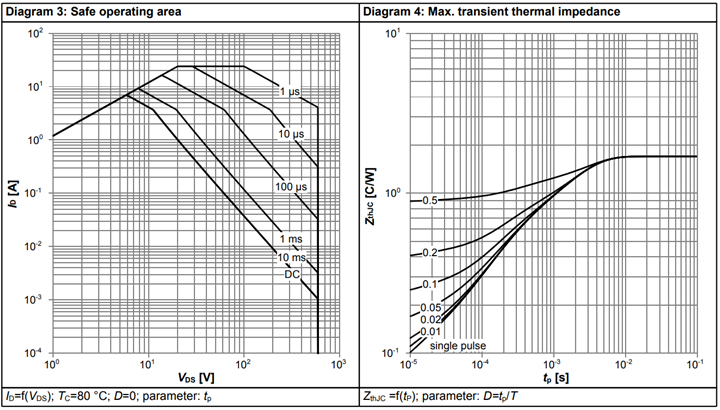To extract the values from the diagrams, you should use a software of your choice. Extracting the data points manually is not recommended as it may cause errors.

1. Derating for different pulse times

The diagram above is an example of the result of derating the SOA curves to different pulse times.

For this, calculate the curves from points A to D. A 62.5-µs pulse time is used in the following example.

## 1.1 RDSON limited line

The Rdson limited line offset and angle are the same for all conditions except for its length. Thus, we have:

Point A: 1 V/1.2 A.

## 1.2 Package limited line

In this example, the power limit is greater than the package limit, so there is no package limited line. If a package limited line is present, draw the line right at the maximum current rating of the device, in the same manner as in the original SOA. The length of the line will be set by Point B, which is calculated in the next section.

## 1.3 Maximum power limit

Point B is set by the maximum power limited by the Tjmax of the device. The maximum power changes depending on the pulse width. This is due to the Zth (thermal impedance) of the device increasing with the pulse width.

You can extract Zth from the max transient thermal impedance diagram. Note that you should use the tool of your choice to minimize errors during extraction.

For a pulse length of 62.5 µs, you can find that Zth is 0.23°C/W. With this, you can calculate the maximum power that can be dissipated with: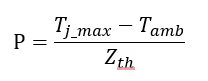Now, choose the point of the Rdson limit line, which would satisfy the following equation: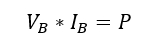In this example, P is equal to 304 W and therefore:

Point B: 19.3 V/15.77 A

Looking back at the SOA diagram, note that regardless of the pulse times, point C is given at the same current point. This is helpful for the derating, as you can use the same formulas as in point B.

The dissipated power is the same; you already know the current (example is 3.5 A). Divide 304 W by 3.5 A to get Point C:

Point C: 87 V/3.5 A

## 1.4 Thermal stability limit

The thermal stability line is defined by the secondary breakdown phenomenon. This line changes depending on the technology being used, which means that it must be derived experimentally.

For derating it, you can derive Point D by using the slope of the line as a guide. Therefore, create a parallel line to this limit line, which starts at point C and ends in Point D.

You already have the x and y coordinates of Point C, as well as the x coordinate of Point D, which is the maximum rated blocking voltage of the device. As the diagram is in logarithmic scale, use the following to obtain the slope of the closest limit line: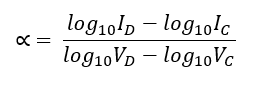With the slope, calculate Id as follows: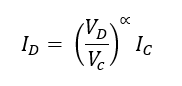For this example, take the 100-µs line as reference. Thus, Vc = 62 V, Ic = 3.72 A, Vd = 600 V, Id = 0.033 A.

The resulting slope is α = –2.07. With this, the resulting Point D coordinates are:

Point D : 600V/0.064A

In conclusion, you can derate the SOA diagram lines for your custom pulse times.

575 ViewsModeratorModerator

Thanks for sharing this, It will be useful information.EmployeeEmployee

Hello,

nice article however you are not explaining how to derate for different ambient or case temperatures.

In your article you refer to a SOA diagram with TC=80degC, most MOSFET have the SOA at 25degC.

It would be great to add a 2nd paragraph Derating for different ambient/case temperature.

Thanks